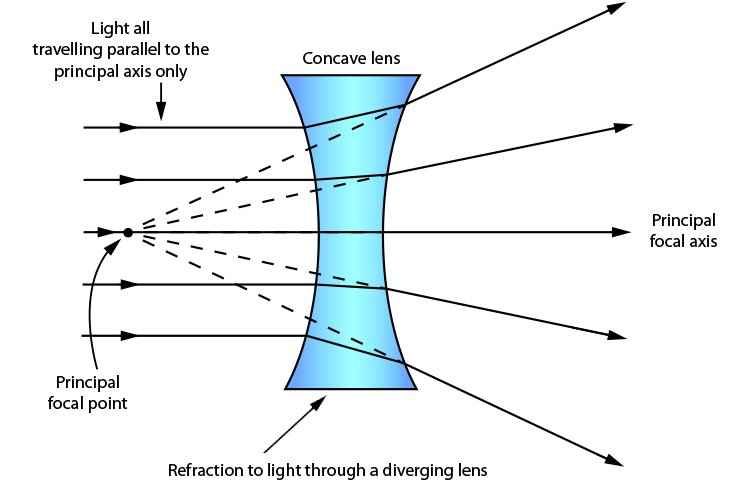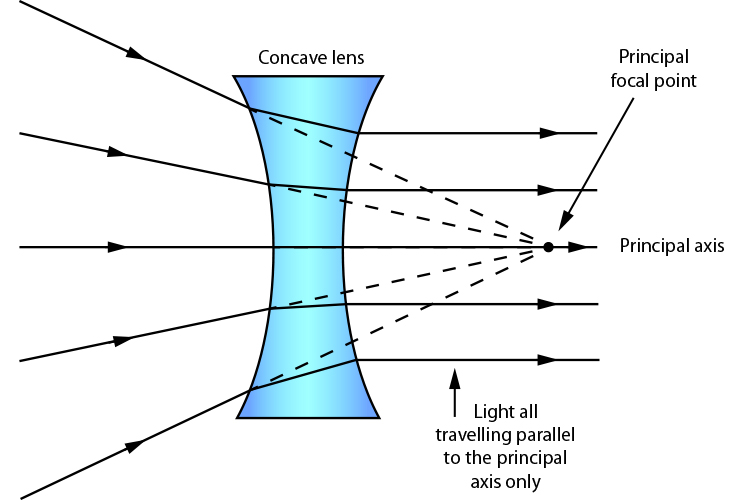# Concave lens – Principal focal point (two of them)

The most important characteristics of a lens is its principal focal point. We know the term principal axis and this gives you a clue that there is a point on the principal axis where light will travel in line with the focal point (principal focal point).

The word shouldn't perhaps be focal; the term divergence point would be a better description of the principal focal point.

But be careful because this only applies to light that is passing parallel to the principal axis.When light rays parallel to the principal axis pass through a concave lens they appear to diverge from one point called the principal focal point.

Unlike with the convex lens, this ray of light is traced back through the principal focal point on the side where the rays came from.

This part of the ray is virtual and is drawn with a dotted line because the light never really goes there.

However, a concave lens has two spherical surfaces and therefore it has two principal focal points.

The second principal focal point is that point where any light ray travelling towards the focal point on the way to the lens will refract through the lens and travel parallel to the principal axis.